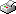Print This Page

Bertil Wegmann

-- licentiate thesis --

##### Second Price Common Value Auctions and Bayesian Inference in Ebay Auctions

Abstract
Second price common value auctions is the topic of this thesis. Estimation of such auctions are technically challenging and equilibrium bid functions in these settings are in general complex and not easy to analyze. In Paper 1 we derive closed form approximations of the bid function for two empirically important models. The approximate bid functions can be evaluated directly without time consuming numerical integration. This is crucial for speeding up likelihood/Bayesian estimations on auction data. In Paper 2 we explore the determinants of bidder and seller behaviour by modelling eBay auctions as independent second price common value auctions, and assume a similar (the same in Paper 1) hierarchical Gaussian valauation structure as in Bajari and Hortacsu (2003). We use an efficient Bayesian variable selection algorithm to assess the importance of the model's covariates. The good performance of the algorithm is documented on both real and simulated data. An important result of Paper 2 is the nearly identical inferences for the approximate bid function in Paper 1 with the exact bid function, which gives much faster and numerically more stable evaluations of the likelihood function. We apply the methodology to simulated data and to a carefully collected dataset of 1000 coin auctions at eBay. The structural estimates are reasonable, both in sign and magnitude, and the model fits the data well. Finally, we document good out-of-sample predictions from the estimated model.

Keywords: Closed form solution, Bid approximation, Normal valuations, Markov Chain Monte Carlo, Variable selection, Internet auctions.